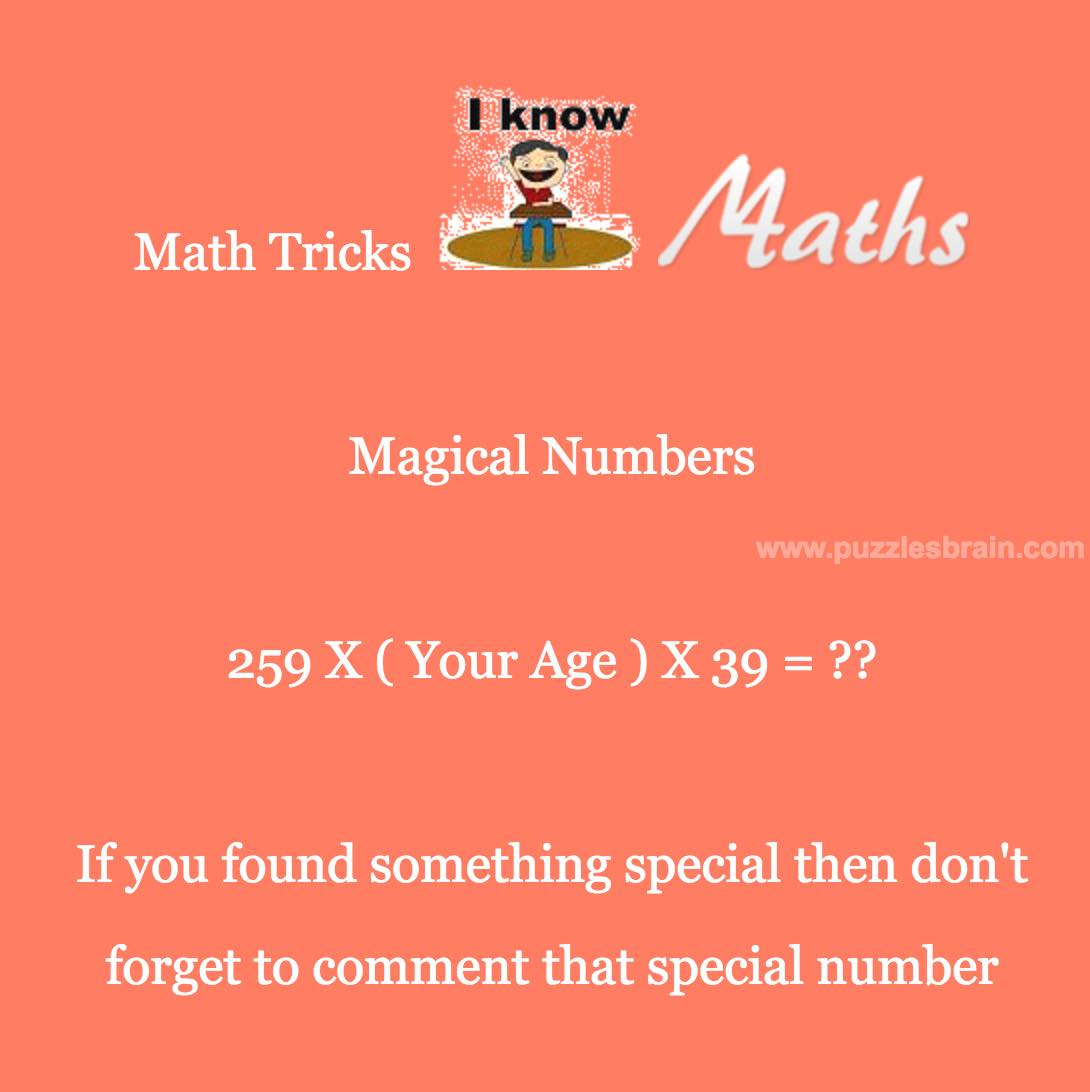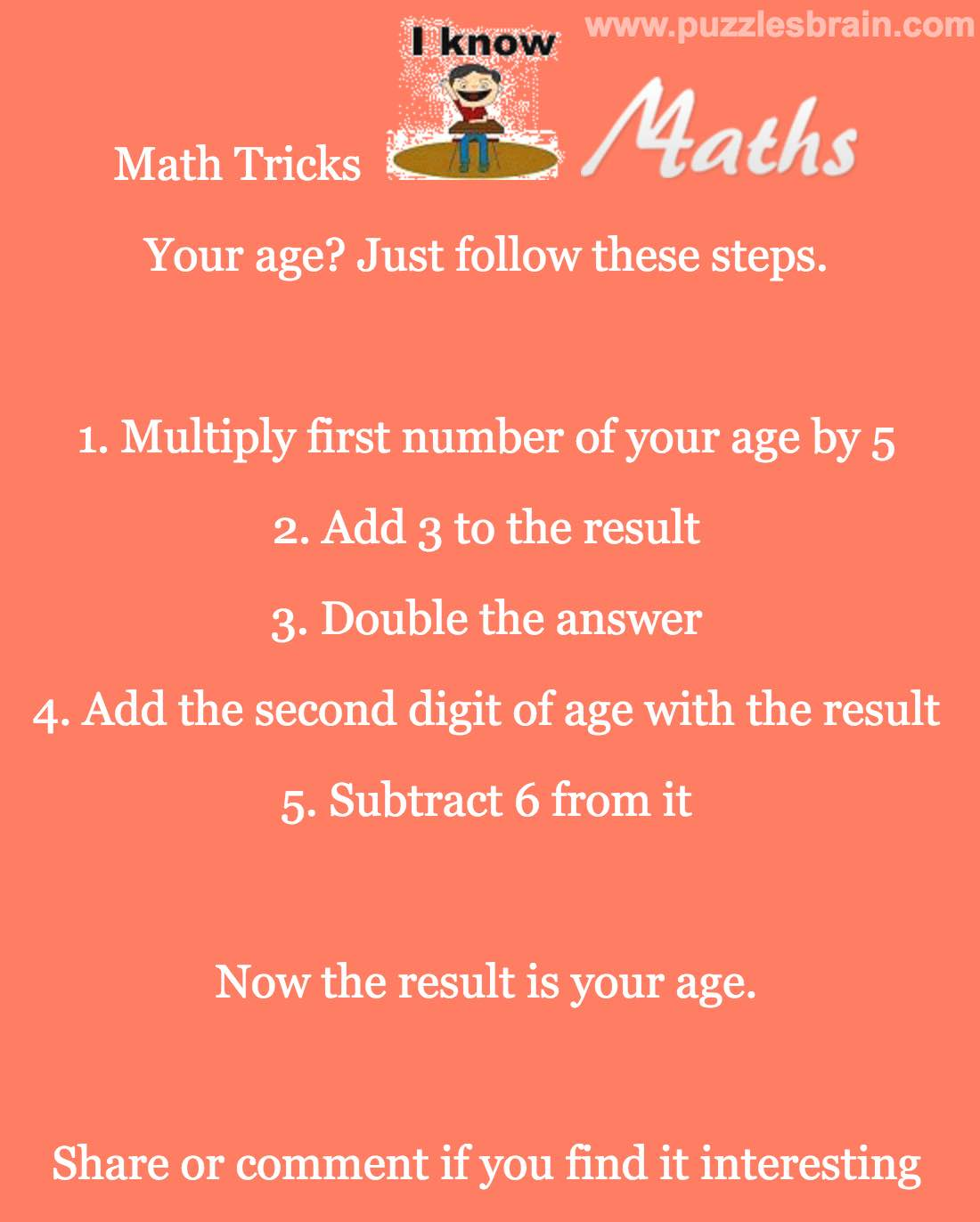Categories

# Best Math Tricks Ever

## Best Math Tricks Ever

Cool Math Trick – Try 259 x (your age) x39

You will get a special number.Magical Numbers:

259 X (Your Age) X 39 = ??

If you found something special then don’t forget to comment that special number. Share this post with your friends and family.

I can tell Number – Guess any number and I will tell the answer

Cool Math number tricks

1. Think of any number.
2. Subtract 1 from the number you thought of
3. Multiply that number to 3
5. Divide the result by 3
7. Subtract the original number you thought of from resultCool Math Trick to tell Age:

You age? Just follow these steps:

1. Multiple first number your age by 5
2. Add 3 to the result
4. Add the second digit of age with the result
5. Subtract6 from it

Now the result is your age.Share this post or comment if you find these tricks interesting. Also, like our FaceBook Page for more puzzles, Brainteasers and riddles.